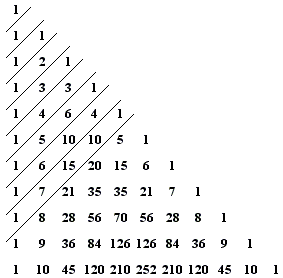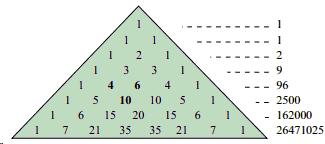# Pascal’s Triangle

Pascal’s triangle is filled with a wealth of interesting patterns, something I never learnt during secondary school when first introduced to this mathematical object. In this post, inspired by a Numberphile video, I want to show you some of these patterns that you might have never seen before.

Firstly, what is Pascal’s Triangle?

Pascal’s triangle is a triangular array of the binomial coefficients.To construct Pascal’s triangle, start with the two top rows, which are 1 and 1 1. To find any number in the next row, add the two numbers above it.At the beginning and end of each row, where there is only one number above, write 1.

### Symmetric

Pascal’s triangle is symmetric, a fact which follows directly from the formula for the binomial coefficient:It can also be seen by how the triangle is constructed, i.e. “by the interpretation of the entries as the number of ways to get from the top to a given spot in the triangle“.

### Sum of entries in row n equals 2n

This fact can be proved by induction. The main point of the argument is that each entry in row n is added to two entries below. This gives us:Hence, the sum of entries in row n+1 is twice the sum of entries in row n.

### Hockey Stick Pattern

In Pascal’s words: “In every arithmetical triangle each cell is equal to the sum of all the cells of the preceding row from its column to the first, inclusive”. This is easier to explain using a diagram:Mathematically, this can be proved by induction and is denoted in the following way:### Fibonacci Numbers

To see this, let us first rearrange Pascal’s triangle.The successive Fibonacci numbers are the sums of the entries on the marked diagonals:

1 = 1

1 = 1

2 = 1 + 1

3 = 1 + 2

5 = 1 + 3 + 1

8 = 1 + 4 + 3

13 = 1 + 5 + 6 + 1

etc.

### e

Recently, the Harlan brothers highlighted that e is hidden in Pascal’s triangle. This was discovered by considering products rather than sums.Denoting Sn as the product of the terms in the nth row, as n tends to infinity, we find that:### Catalan Numbers

Catalan numbers can be found in Pascal’s triangle in a few ways, for example:

• If you take each ‘middle’ element and subtract its adjacent entry, you get a Catalan number.• If you take a middle element and divide it by its position in the list of middle terms (e.g. divide the 5th middle term by 5), you will get a Catalan number.

There are many more patterns, and I encourage you to find out more about them! M x

## One thought on “Pascal’s Triangle”

1.dnfibmi8an@gmail.com says:

best security system 2018

Like